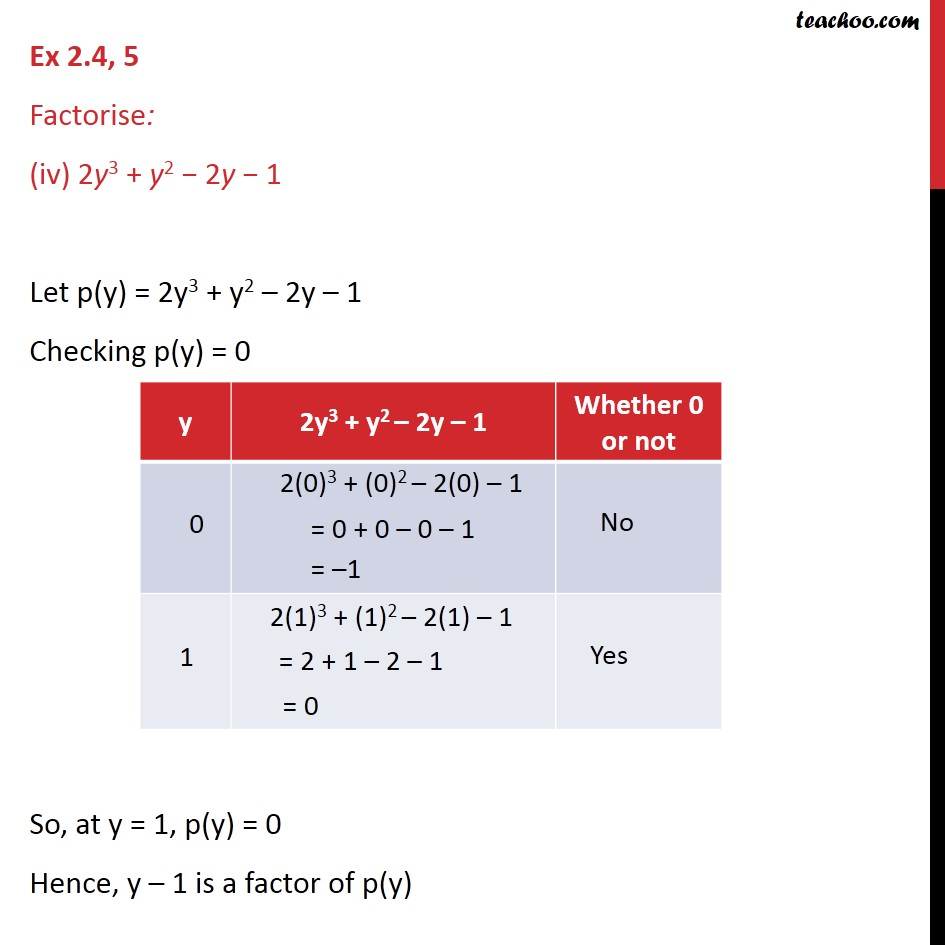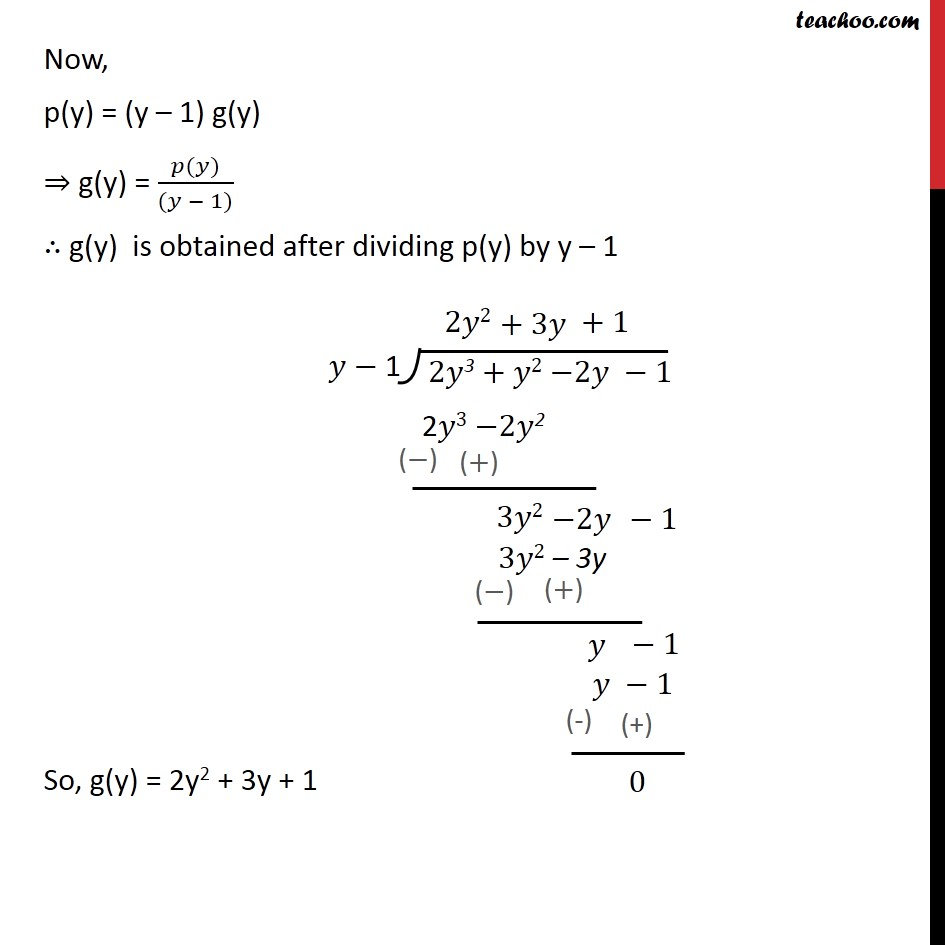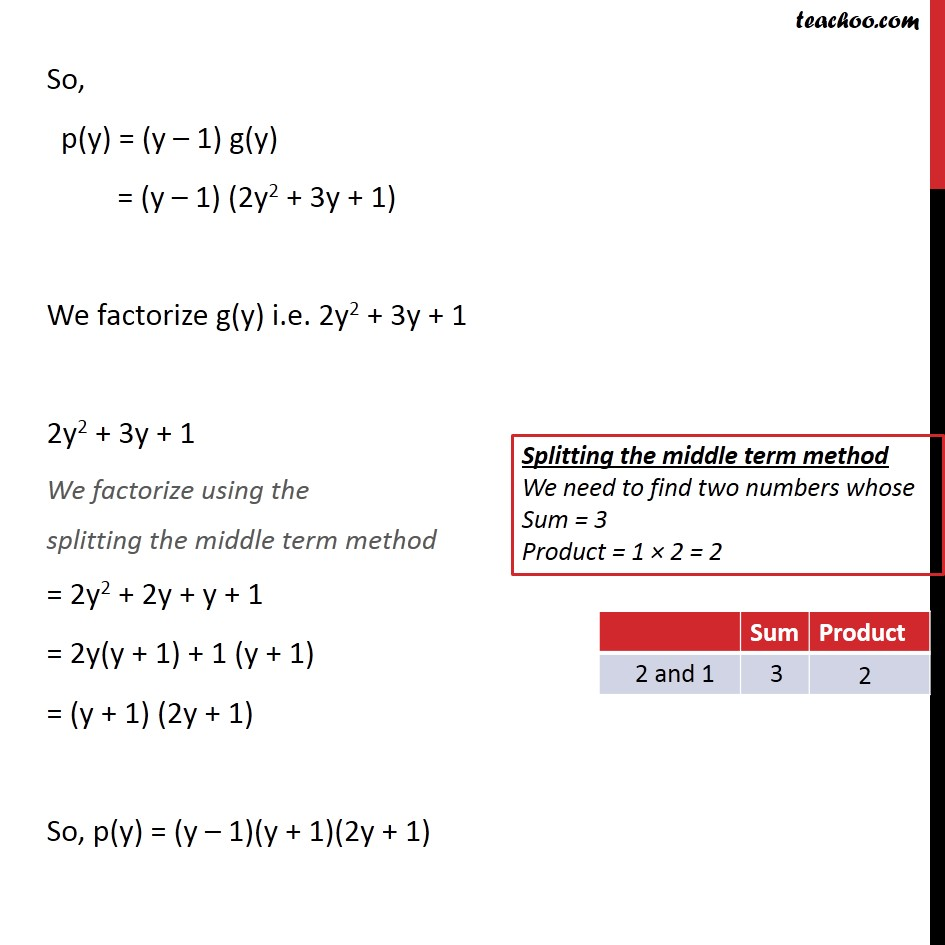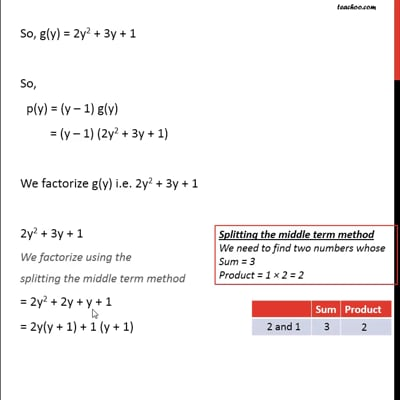Ex 2.4

Chapter 2 Class 9 Polynomials
Serial order wiseThis video is only available for Teachoo black users

Introducing your new favourite teacher - Teachoo Black, at only ₹83 per month

### Transcript

Ex 2.4, 5 Factorise: (iv) 2y3 + y2 − 2y − 1 Let p(y) = 2y3 + y2 – 2y – 1 Checking p(y) = 0 So, at y = 1, p(y) = 0 Hence, y – 1 is a factor of p(y) Now, p(y) = (y – 1) g(y) ⇒ g(y) = (𝑝(𝑦))/((𝑦 − 1)) ∴ g(y) is obtained after dividing p(y) by y – 1 So, g(y) = 2y2 + 3y + 1 So, p(y) = (y – 1) g(y) = (y – 1) (2y2 + 3y + 1) We factorize g(y) i.e. 2y2 + 3y + 1 2y2 + 3y + 1 We factorize using the splitting the middle term method = 2y2 + 2y + y + 1 = 2y(y + 1) + 1 (y + 1) = (y + 1) (2y + 1) So, p(y) = (y – 1)(y + 1)(2y + 1)